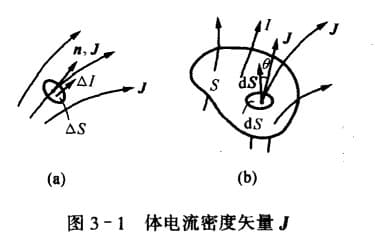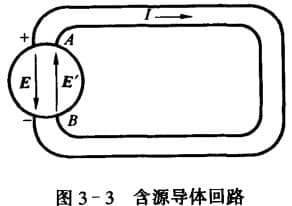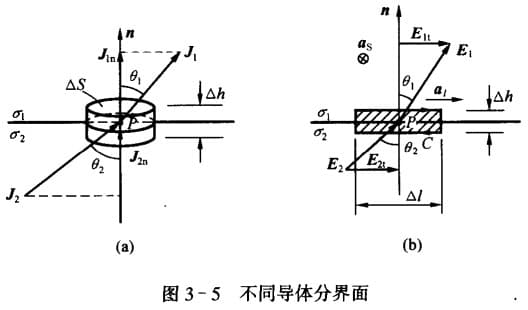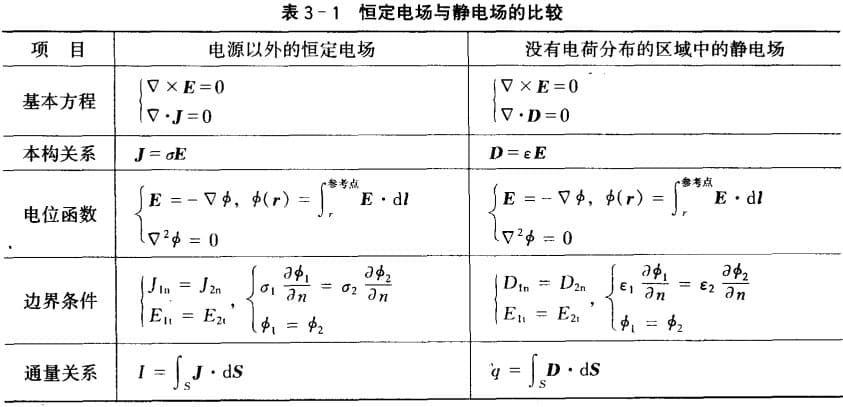# 电流

## 体电流密度矢量

$\bd{n}$ 是正电荷运动的方向（电流方向），$\bd{S}$ 是在该点上垂直于 $\bd{n}$ 的面元。我们可以用 $\bd{J}$ 的矢量线来描绘电流场。## 面电流密度矢量

$\hat{n}$ 是电流方向，$\Delta l$ 是垂直于 $\hat{n}$ 的线元。也可以用面电荷密度 $\rho_{vs}$ 与速度 $\bd{v}$ 来表示：

# 欧姆定律

• 微分形式适用于任何连续导电媒质、任何传导电流；
• 积分形式指适用于均匀媒质、恒定电流；# 分界面上的边界条件# 电导与接地电阻

• 用电流计算
• $假设 I\rightarrow \vec{E}=\frac{\vec{J}}{\sigma}\rightarrow $$U=\int \vec{E}\cdot\dif \vec{l}\rightarrow G=\frac{I}{U} • 假设 U\rightarrow \vec{J}=\sigma \vec{E}\rightarrow$$I=\int \vec{J}\cdot\dif \vec{S}\rightarrow G=\frac{I}{U}$
• 静电比拟法
• $\dfrac{C}{G}=\dfrac{Q/U}{I/U}=\dfrac{Q}{I}=$$\dfrac{\oint_s \vec{D}\cdot\dif\vec{S}}{\oint_s \vec{J}\cdot\dif\vec{S}}=\dfrac{\varepsilon}{\sigma}$

# 静电场与恒定电场比较1. 传导电流：传导电流中的带电微粒(如金属中的自由电子、电解质溶液中的正负离子、气体中的离子和电子)在电场作用下，在导体内部做定向运动而形成的电流。（百度百科）